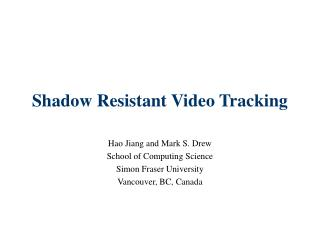DownloadDownload PresentationTélécharger la présentation- - - - - - - - - - - - - - - - - - - - - - - - - - - E N D - - - - - - - - - - - - - - - - - - - - - - - - - - -
##### Presentation Transcript

1. Shadow Resistant Video Tracking Hao Jiang and Mark S. Drew School of Computing Science Simon Fraser University Vancouver, BC, Canada

2. Problem Statement We want to realize this! Traditional Contour Tracking based on motions

3. Outline • We present an invariant image model. We study how to project an image to an invariant space, such that the shadow can be greatly attenuated. • We present two new external forces to the snake model and present an chordal snake model to deal with object tracking in cluttering environment. • The first external force is based on predictive contour • The chordal constraint based on a new shape descriptor • Results and conclusion

4. Invariant Image n Planckian Lighting ai x Lambertian Surface For narrow band Sensors: The responses:

5. ref-2 Considering 3-sensor cameras, = R, = G, = B Let r=log(R/G) , b=log(B/G) We get, b ref-1 The slope is determined by the camera sensors Lighting … Material r

6. Invariant Image Generation (log(r/g), log(b/g)) Projection (r,g,b) b Invariant Image Generation Camera characteristic orientation o r

7. Camera Calibration Take image of one scene under different lightings Shift the center of the log-log ratios corresponding to each material to the origin Stack the log-ratio vectors of each material into a matrix A and do SVD A=UDV’ Camera Orientation= V(:,1) Characteristic Orientation of Canon ES60

8. For Real Image Original image Invariant image

9. Inertia Snake Tracking I • A predictive contour constraint Inertia Term If we choose quadratic norm for E(.,.) the Eular Equation, By introducing a artificial parameter t, the equation can be solved by PDE

10. A Chordal Constraint • Now we further introduce a second constraint to maintain the solidness of the shape of the contour by maintaining the value of a shape descriptor. • The shape discriptor is defined as d(s, )=||X(s)-X(s+ )|| where s in is the normalized length from one point on the contour. Apparently, d(x, ) is periodic for both s and . s 1 1 0

11. The Shape descriptor s 1 0 1 The similarity of contour X and Y is, In frequency domain

12. The Chordal Snake Here we use a simple version d(s)=||X(s)-X(s+1/2)|| The variational problem is Where Y(s) is an accessory contour, d(s) is the calculated from last video frame. The corresponding reaction-diffusion PDE is:

13. The Chordal Snake Now we set Y0(s)= X0(s+1/2), D0(s)= C0(s+1/2). It is not difficult to prove the following Lemma and theorem. Lemma: If Y(s,t1)= X(s+1/2,t1), D(s,t1)= C(s+1/2,t1) then Y(s,t)= X(s+1/2,t) for any t>= t1 Theorem: Given the initial conditions of Y0(s)= X0(s+1/2), D0(s)= C0(s+1/2), we have, Predictive Constraint Shape descriptor constraint

14. Chordal Snake Tracking II Chordal constraint Predictive contour Features Smoothed predictive contour Previous contour Real object boundary Initial contour

15. The System Motion Detection Affine Motion Estimation Fn Motion Map Fn-1 Invariant Image warping GVF Invariant Image Motion Detection Cn-1 Contour Prediction External Force Init Contour Pred Contour Chordal Model Cn Inertia Snake Tracking

16. An Example Initial Contour Prediction Contour

17. Experiment Result Two successive frames Motion map in original color space Motion map in invariant color space

18. Experiment Result Two successive frames Motion map in original color space Motion map in invariant color space

19. Traditional Snake Model Frame 1 Frame 2 Frame 3 Frame 4 Frame 5 Frame 6 Frame 7 Frame 8 Frame 9

20. Tracking Result Ball Sequence Hand Sequence Baby Sequence

21. Conclusion • We present scheme to get shadow invariant image. • We present a much more robust snake model. • The proposed method can work well even though there is strong distracting shadows • Current framework can be easily extended to the cases when the object is passing casting shadows • Future Work • Study scheme to deal with tracking in high dynamic range environment • Study shadow resistant method for active appear model

22. Thank You! Q&A